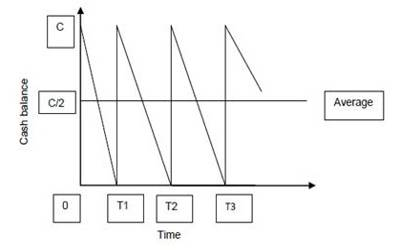# Baumol Model and it’s Assumption

Baumol Model and its Assumption –

Most firms try to minimize the sum of the cost of holding cash and the cost of converting marketable securities to cash. Baumol model of cash management helps in determining a firm’s optimum cash balance under certainty.

Baumol’s cash management model helps in determining a firm’s optimum cash balance under certainty. As per the model, cash and inventory management problems are one and the same. It is extensively used and highly useful for the purpose of cash management. There are certain assumptions that are made in the model. They are as follows:

1. The firm is able to forecast its cash requirements with certainty and receive a specific amount at regular intervals.
2. The firm’s cash payments occur uniformly over a period of time i.e. a steady rate of cash outflows.
3. The opportunity cost of holding cash is known and does not change over time. Cash holdings incur an opportunity cost in the form of opportunity foregone.
4. The firm will incur the same transaction cost whenever it converts securities to cash. Each transaction incurs a fixed and variable cost.
5. The company is aware of the opportunity cost required for holding cash. It should stay the same for a considerable length of time.

For example, let us assume that the firm sells securities and starts with a cash balance of C rupees. When the firm spends cash, its cash balance starts decreasing and reaches zero. The firm again gets back its money by selling marketable securities. As the cash balance decreases gradually, the average cash balance will be: C/2. This can be shown in the following figure:The firm incurs a cost known as holding cost for maintaining the cash balance. It is known as opportunity cost, the return inevitable on the marketable securities. If the opportunity cost is k, then the firm’s holding cost for maintaining an average cash balance is as follows:

Holding cost = k (C/2)

Whenever the firm converts its marketable securities to cash, it incurs a cost known as a transaction cost. The total number of transactions in a particular year will be total funds required (T), divided by the cash balance (C), i.e., T/C. The assumption here is that the cost per transaction is constant. If the cost per transaction is c, then the total transaction cost will be:

Transaction cost = c (T/C).

The total annual cost of the demand for cash will be: Total cost = k (C/2) + c (T/C)